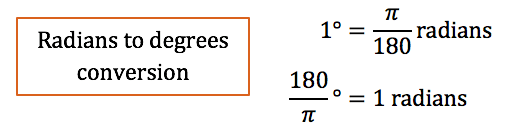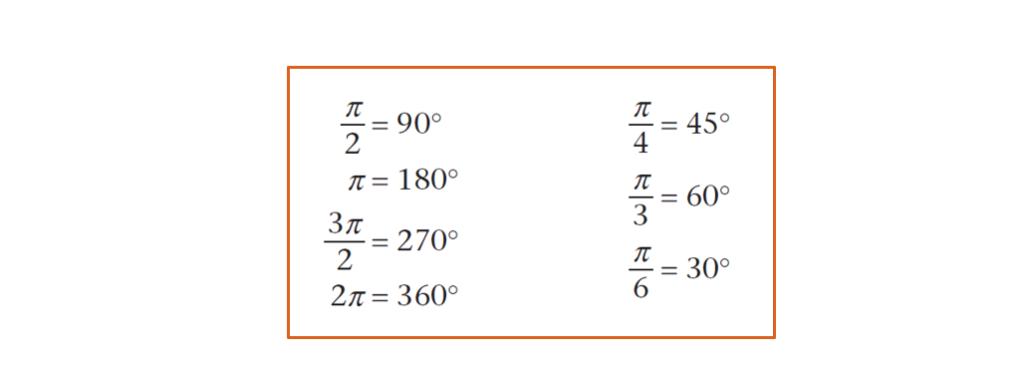PRIMARY

3 - 6

INTERMEDIATE

7 - 8

SECONDARY

9 - 11

Home

Theory & Practice Questions.

## Theory

One radian is the angle that an arc of 1 unit subtends at the centre of a circle of radius 1 unit.π^c = 180°

## Relationship between radian measure and degreesCircumference of the circle with radius 1 unit is given by:

C = 2πr

= 2π(1)

= 2π

T

The arc length of the whole circle is .

There are radians in a whole circle.

But there are 360° in a whole circle (angle of revolution).

So 2π^c = 360°

π^c = 180°

To change from radians to degrees: multiply by 180/πNote: Special measure you will use regularly include -## Practice Question

1. Convert 5π/6 radians into degrees.
##### Solution
1. To convert radians into degrees:Therefore:

5π/6 radians = 180/π° x 5π/6

Simplyfying:

=900π/6π°

=150°

##### Want a FREE question and answer worksheet?